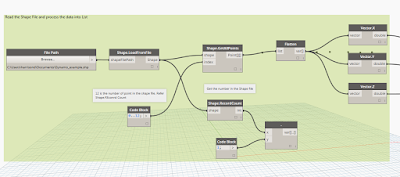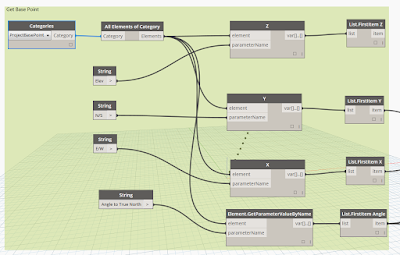# InfraBIM - Infrastructure BIM & GIS

BIM works flows of using Autodesk products, such as Infraworks, Civil 3d, Map 3d, Recap Pro and Navisworks that makes my life easier.

## Tuesday, January 26, 2016

### GIS into Revit using Dynamo

Revit does not have a import function for a GIS ESRI Shapefile. There are multiple work follows of using MAP 3d or Civil 3d and then importing a .dwg into Revit. Dynamo and the package of GIS  now allows the import function of points, curves and Polygons. The current Package on Dynamo only imports the geometry with in the Shape file and not attributed information, this is currently not available with in the GIS package.

The image of the Dynamo script below uses GIS package in Dynamo to read the shape file and insert point families into Revit. As noted before only the point and curve geometry is import into Revit, no attributed data. The Points are matrix transposes the point to suit the Revit base point to insert point base family in the correct location.The above appears to be complex set of node but real are just a simple connection of node that performs a simple task;

1. Read the ESRI Shapefile
2. Process the ESRI Shapefile into list
3. Get the Project Base Point and rotation
4. Matrix transform the point form the Project base point
5. Insert Point family from the transformed base pointsThe above image is a group of nodes to read the ESRI Shapefile

The process in the above image includes to read the entries of the points with in the Shape file. The result of the list on points is need to be inserted into the Code block to list

Group of nodes to get the project base Point.The next node group is thanks to Wikipedia! If you can under stand Revit shared coordinates well try think of it backwards!!!

Revit's project base point is the mathematical set out point of the graphical data base. The project base point removes large numbers from the data base and makes the math simple. I actually don't know how to write it in words but to put it simple if you had the option of x =235982030, y=230923092, Z=14000 vs  x=1, y =1, z = 1 for every point as a reference point which one would you chose?  Well Revit makes it simple by using 1,1,1 and tell base point is the large number which is multiplied by linear algebra.  To get the reverse into Revit from Dynamo you need to take the points that you have and Matrix transform them from the Project base point and rotation to true north. Year 12 linear algebra, comes to mind.

Refer to the Wikipedia for Matrix transform https://en.wikipedia.org/wiki/Transformation_matrix

### Rotation

For rotation by an angle θ clockwise about the origin the functional form is$x' = x \cos \theta + y \sin \theta$ and$y' = -x \sin \theta + y \cos \theta$. Written in matrix form, this becomes:$\begin{bmatrix} x' \\ y' \end{bmatrix} = \begin{bmatrix} \cos \theta & \sin\theta \\ -\sin \theta & \cos \theta \end{bmatrix} \begin{bmatrix} x \\ y \end{bmatrix}$

Simple math but complex to get at the start.

Once the Points have been reversed transform then can been used as points with in Revit

Download the updated dynamo script Link
Download the shape file Example Link